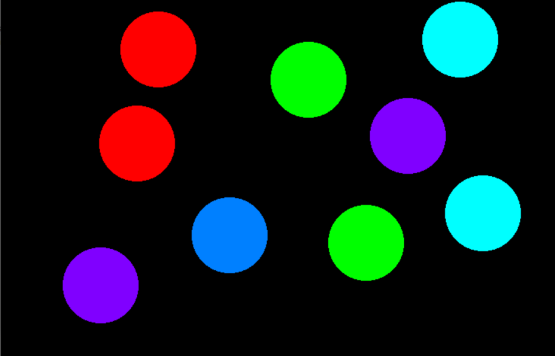# Program to draw circles using mouse moves in OpenGL

• Last Updated : 16 Jul, 2021

In this article, the task is to draw circles using a single mouse click in OpenGL.

OpenGL: OpenGL is a cross-language, cross-platform API for rendering 2D and 3D Vector Graphics. It will make a lot of design as well as animations using this.

• Create a circle anywhere on the console using a single left mouse click and the coordinates of the center of the circle created depends on the position of your click.
• To change the color of the circle, simply right-click on the mouse.
• After performing all operations jump out of the program by simply pressing the Esc key on the keyboard.

Approach: The idea is to use the below inbuilt function to draw the circle using single click in OpenGL:

• glMatrixMode(GL_PROJECTION): This function sets the current matrix to projection.
• glLoadIdentity(): The function is used to multiply the current matrix by identity matrix.
• gluOrtho2D(0.0, 800.0, 0.0, 600.0): It sets the parallel(orthographic) projection of the full frame buffer.
• glutCreateWindow(“Circle Creation on mouse click”): Creates the window as specified by the user as above.
• glClearColor(0, 0, 0, 0): It sets the background color.
• glClear(GL_COLOR_BUFFER_BIT): It clears the frame buffer and set values defined in glClearColor() function call.
• glutDisplayFunc(display): It links the display event with the display event handler(display).
• glutMouseFunc(mouse): Mouse event handler.
• glutKeyboardFunc(keyboard): Keyboard event handler.
• glutMainLoop(): This function loops the current event.

Below is a C++ program that implements onClick functionality in OpenGL:

## C++

 `// C++ program to implement onClick` `// functionality in OpenGL to draw` `// a circle` `#include ` `#include ` `#include ` `#include ` `#define xpix 500` `#include ` `using` `namespace` `std;`   `float` `r, g, b, x, y;` `bool` `flag = ``true``;` `int` `counter = 0;`   `// Function works on mouse click` `void` `mouse(``int` `button, ``int` `state,` `           ``int` `mousex, ``int` `mousey)` `{` `    ``if` `(button == GLUT_LEFT_BUTTON` `        ``&& state == GLUT_DOWN) {` `        ``flag = ``true``;` `        ``x = mousex;` `        ``y = 600 - mousey;` `    ``}`   `    ``// Change color of circle` `    ``else` `if` `(button == GLUT_RIGHT_BUTTON` `             ``&& state == GLUT_DOWN) {` `        ``if` `(counter > 4) {` `            ``counter = 0;` `        ``}`   `        ``counter++;`   `        ``// Redisplay` `        ``glutPostRedisplay();` `    ``}` `}`   `// Function that exits from program` `void` `keyboard(unsigned ``char` `key,` `              ``int` `x, ``int` `y)` `{` `    ``switch` `(key) {` `    ``case` `27:` `        ``glutHideWindow();` `    ``}` `}`   `// Function to draw the circle` `void` `display(``void``)` `{` `    ``float` `angle_theta;` `    ``if` `(counter == 1) {` `        ``glColor3f(1, 0, 0);` `    ``}` `    ``else` `if` `(counter == 2) {` `        ``glColor3f(0, 1, 0);` `    ``}` `    ``else` `if` `(counter == 3) {` `        ``glColor3f(0, 1, 1);` `    ``}` `    ``else` `if` `(counter == 4) {` `        ``glColor3f(0.5, 0, 1);` `    ``}` `    ``else` `if` `(counter == 5) {`   `        ``glColor3f(0, 0.5, 1);` `    ``}`   `    ``// Matrix mode` `    ``glMatrixMode(GL_PROJECTION);` `    ``glLoadIdentity();`   `    ``// Given the coordinates` `    ``gluOrtho2D(0.0, 800.0,` `               ``0.0, 600.0);`   `    ``// If the flag is true` `    ``if` `(flag) {`   `        ``// Begin the pointer` `        ``glBegin(GL_POLYGON);`   `        ``// Iterate through all the` `        ``// 360 degrees` `        ``for` `(``int` `i = 0; i < 360; i++) {`   `            ``// Find the angle` `            ``angle_theta = i * 3.142 / 180;` `            ``glVertex2f(x + 50 * ``cos``(angle_theta),` `                       ``y + 50 * ``sin``(angle_theta));` `        ``}`   `        ``// Sets vertex` `        ``glEnd();` `    ``}`   `    ``// Flushes the frame buffer` `    ``// to the screen` `    ``glFlush();` `}`   `// Driver Code` `int` `main(``int` `argc, ``char``** argv)` `{`   `    ``glutInit(&argc, argv);` `    ``glutInitWindowSize(800, 600);` `    ``glutInitWindowPosition(100, 100);` `    ``glutInitDisplayMode(GLUT_SINGLE | GLUT_RGB);`   `    ``// Creates the window as` `    ``// specified by the user` `    ``glutCreateWindow(``"Circle Creation "` `                     ``"on mouse click"``);`   `    ``// Sets the background color` `    ``glClearColor(0, 0, 0, 0);`   `    ``// Clears the frame buffer` `    ``glClear(GL_COLOR_BUFFER_BIT);`   `    ``// Links display event with the` `    ``// display event handler(display)` `    ``glutDisplayFunc(display);`   `    ``// Mouse event handler` `    ``glutMouseFunc(mouse);`   `    ``// Keyboard event handler` `    ``glutKeyboardFunc(keyboard);`   `    ``// Loops the current event` `    ``glutMainLoop();` `}`

Output:My Personal Notes arrow_drop_up
Recommended Articles
Page :